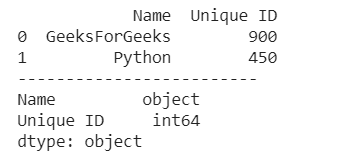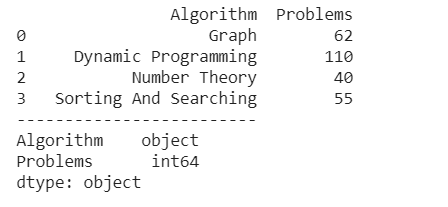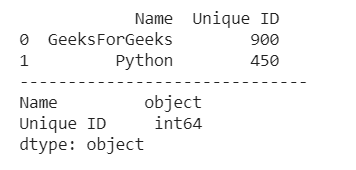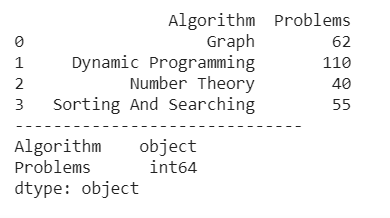Related Articles

# How to Convert String to Integer in Pandas DataFrame?

• Difficulty Level : Easy
• Last Updated : 26 Sep, 2021

Let’s see methods to convert string to an integer in Pandas DataFrame:

Method 1: Use of Series.astype() method.

Attention geek! Strengthen your foundations with the Python Programming Foundation Course and learn the basics.

To begin with, your interview preparations Enhance your Data Structures concepts with the Python DS Course. And to begin with your Machine Learning Journey, join the Machine Learning - Basic Level Course

Syntax: Series.astype(dtype, copy=True, errors=’raise’)

Parameters: This method will take following parameters:

• dtype: Data type to convert the series into. (for example str, float, int).
• copy: Makes a copy of dataframe/series.
• errors: Error raising on conversion to invalid data type. For example dict to string. ‘raise’ will raise the error and ‘ignore’ will pass without raising error.

Return: Series with changed data type.

One of the most effective approaches is Pandas astype(). It is used to modify a set of data types. The columns are imported as the data frame is created from a csv file and the data type is configured automatically which several times is not what it should have. For instance, a salary column may be imported as a string but we have to convert it into float to do operations.

Example 1:

## Python3

 `# import pandas library``import` `pandas as pd` `# dictionary``Data ``=` `{``'Name'``: [``'GeeksForGeeks'``,``'Python'``],``          ``'Unique ID'``: [``'900'``,``'450'``]}` `# create a dataframe object``df ``=` `pd.DataFrame(Data)` `# convert string to an integer``df[``'Unique ID'``] ``=` `df[``'Unique ID'``].astype(``int``)` `# show the dataframe``print` `(df)``print``(``"-"``*``25``)` `# show the data types``# of each columns``print` `(df.dtypes)`

Output :Example 2:

## Python3

 `# import pandas library``import` `pandas as pd` `# dictionary``Data ``=` `{``'Algorithm'``: [``'Graph'``, ``'Dynamic Programming'``,``                      ``'Number Theory'``,``                      ``' Sorting And Searching'``],``        ` `          ``'Problems'``: [``'62'``, ``'110'``, ``'40'``, ``'55'``]}` `# create a dataframe object``df ``=` `pd.DataFrame(Data)` `# convert string to integer``df[``'Problems'``] ``=` `df[``'Problems'``].astype(``int``)` `# show the dataframe``print` `(df)``print``(``"-"``*``25``)` `# show the data type``# of each columns``print` `(df.dtypes)`

Output :Method 2: Use of  pandas.to_numeric () method.

Syntax: pandas.to_numeric(arg, errors=’raise’, downcast=None)

Parameters: This method wil take following parameters:

• arg: list, tuple, 1-d array, or Series.
• errors: {‘ignore’, ‘raise’, ‘coerce’}, default ‘raise’
-> If ‘raise’, then invalid parsing will raise an exception
-> If ‘coerce’, then invalid parsing will be set as NaN
-> If ‘ignore’, then invalid parsing will return the input
• downcast: [default None] If not None, and if the data has been successfully cast to a numerical dtype downcast that resulting data to the smallest numerical dtype possible according to the following rules:
-> ‘integer’ or ‘signed’: smallest signed int dtype (min.: np.int8)
-> ‘unsigned’: smallest unsigned int dtype (min.: np.uint8)
-> ‘float’: smallest float dtype (min.: np.float32)

Returns: numeric if parsing succeeded. Note that return type depends on input. Series if Series, otherwise ndarray.

pandas.to numeric() is one of the widely used methods in order to convert argument to a numeric form in Pandas.

Example 1:

## Python3

 `# import pandas library``import` `pandas as pd` `# dictionary``Data ``=` `{``'Name'``: [``'GeeksForGeeks'``,``'Python'``],``          ``'Unique ID'``: [``'900'``,``'450'``]}` `# create a dataframe object``df ``=` `pd.DataFrame(Data)` `# convert integer to string``df[``'Unique ID'``] ``=` `pd.to_numeric(df[``'Unique ID'``])` `# show the dataframe``print` `(df)``print``(``"-"``*``30``)` `# show the data type``# of each columns``print` `(df.dtypes)`

Output :Example 2:

## Python3

 `# import pandas library``import` `pandas as pd` `# dictionary``Data ``=` `{``'Algorithm'``: [``'Graph'``, ``'Dynamic Programming'``,``                      ``'Number Theory'``,``                      ``' Sorting And Searching'``],``        ` `          ``'Problems'``: [``'62'``, ``'110'``, ``'40'``, ``'55'``]}` `# create a dataframe object``df ``=` `pd.DataFrame(Data)` `# convert strint to an integer``df[``'Problems'``] ``=` `pd.to_numeric(df[``'Problems'``])` `# show the dataframe``print` `(df)``print``(``"-"``*``30``)` `# show the data type``# of each column``print` `(df.dtypes)`

Output :My Personal Notes arrow_drop_up